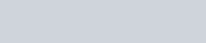ChemTalk

# Graham’s Law of Effusion## Core Concepts

In this tutorial, you will learn about Graham’s Law of Effusion. Additionally, this includes understanding the concepts of the originator, Thomas Graham, and learning the definition of effusion. You will also learn how to use the rate of effusion formula and comprehend the idea of inverse proportion that is significant in using such a formula.

## What is Effusion?

As you begin to understand what Graham’s Law of Effusion is, it is important to know what effusion means. So what is effusion? Effusion is the rate at which gas escapes a container through a small hole into another container. While effusion is the escaping of gas, it is important not to be confused with diffusion. Diffusion refers to the distribution of a substance of higher concentration into an area of low concentration.

### Examples of Diffusion and Effusion

When contrasting the diffusion and effusion of gases, it can be helpful to consider the example of a helium balloon. Helium (He) is commonly used to inflate balloons to allow them to float, but have you noticed balloons deflate over time? This is an example of effusion, and the balloon deflates because lighter helium atoms are able to be effused out of the small holes present in the rubber. This phenomenon is also present in the air pressure of tires, as the tire continues to be used air molecules are able to effuse out of the tires through microscopic holes.Helium balloons shrink due to the effusion of the helium through small holes

In diffusion, the substance of high concentration gets distributed into an area of low concentration. An example of diffusion in real life is a tea bag, when placing a tea bag into a cup of hot water the tea will diffuse out of the tea bag into the hot water resulting in a cup of tea. Another example of diffusion is perfume, if a perfume is sprayed in one corner of a room, the molecules inside the perfume will eventually diffuse to fill the entire room.

## What is Graham’s Law of Effusion?Graham’s Law of Effusion (1848) states that the effusion rate remains inversely proportional to the square root of the molar masses of the gas. As discussed previously, effusion means the rate at which gas escapes from a hole in a container. With inverse proportion, this explains that as the molar mass of a gas increases, the rate of effusion decreases.

### The use of Inverse Proportion in Graham’s Law of Effusion

When understanding that the effusion rate remains inversely proportional to the square root of the molar masses of the gas, it is important to know how inverse proportion works. When discussing proportion in chemistry, we imagine that both values are increasing or decreasing at a consistent rate.

This is exactly the opposite in inverse proportion, as one value increases the other will decrease, and vice versa.

## Units used in the Rate of Effusion EquationTo fully understand the Rate of Effusion Equation, you need to understand all the units it uses. Sometimes, you might not know either the rate of effusion or the molar mass.

Rate A is the Rate of Effusion for the first gas

• The units for Rate A can consist of mol, mL, g, etc. This is typically a mass, over a unit of time, such as m, s, etc.

Rate B is the Rate of Effusion for the second gas

Units used in Rate B are the same for the second gas, consisting of a mass unit over a time unit.

Molar Mass A is the Molar mass of the first gas

• When using or finding the molar masses of the gases in the Rate of Effusion equation, refer to Calculating Molar Mass

Molar Mass B is the Molar mass of the second gas

## Who is Thomas Graham?

Scottish chemist, Thomas Graham (1805-1869), was the pioneer in the effusion of gases. Graham was born in Glasgow, Scotland, and eventually became a student at the University of Glasglow. After completing his undergraduate degree, he continued his studies at the University of Edinburgh where he pursued medicine.

Some of Graham’s most notable work includes his work in the effusion and diffusion of gases. Graham was also a pioneer in dialysis, which is a treatment that removes excessive amounts of water, solutes, and toxins from the blood when the kidneys are unable to.